### Home > CC4 > Chapter 8 > Lesson 8.2.3 > Problem8-123

8-123.
1. If f(x) = 3(2)x, evaluate the expressions in parts (a) through (c) below. Then complete parts (d) and (e). 8-123 HW eTool (Desmos). Homework Help ✎

1. f(−1)

2. f(0)

3. f(1)

4. What value of x gives f(x) = 12?

5. Where does the graph of this function cross the x‑axis? The y‑axis? Use this information to sketch the graph.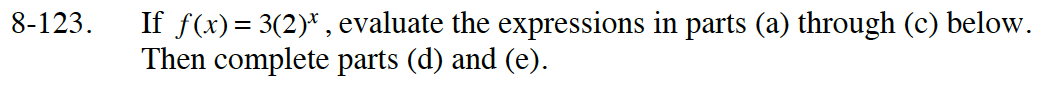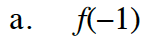Substitute −1 for x and simplify.

f(−1) = 3(2)−1

$=3\cdot\frac{1}{2}$

$\frac{3}{2}$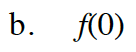Refer to part (a).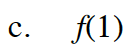Refer to part (a).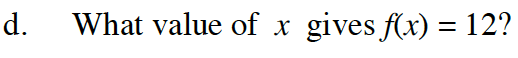Substitute 12 for f(x) and solve for x.

x = 2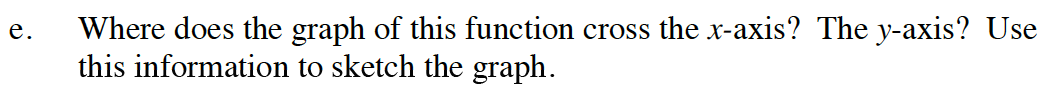A function crosses the x-axis at y = 0, and a function crosses the y-axis at x = 0.

Use the eTool below to find the value of each expression in parts (a) through (c).
Click on the link at right for the full eTool version: Int1 8-123 HW eTool.